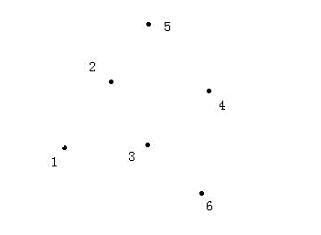Online JudgeProblem SetAuthorsOnline ContestsUser
Web Board
F.A.Qs
Statistical Charts
Problems
Submit Problem
Online Status
Prob.ID:
Register
Authors ranklist
Current Contest
Past Contests
Scheduled Contests
Award Contest
Register
Language:
Linearity
 Time Limit: 3000MS Memory Limit: 65536K Total Submissions: 8724 Accepted: 2011

Description

Alice often examines star maps where stars are represented by points in a plane and there is a Cartesian coordinate for each star. Let the Linearity of a star map be the maximum number of stars in a straight line.For example, look at the star map shown on the figure above, the Linearity of this map is 3, because the star 1, star 2, and star 5 are within the same straight line, and there is no straight line that passes 4 stars.

You are to write a program to find the Linearity of a star map.

Input

Input will contain multiple test cases. Each describes a star map.

For each test case, the first line of the input contains the number of stars N (2 <= N <= 1000). The following N lines describe coordinates of stars (two integers X and Y per line separated by a space, 0 <= X, Y <= 1000). There can be only one star at one point of the plane.

Output

Output the Linearity of the map in a single line.

Sample Input

```5
0 0
2 0
0 2
1 1
2 2
```

Sample Output

`3`

Source

[Submit]   [Go Back]   [Status]   [Discuss]Home PageGo BackTo top